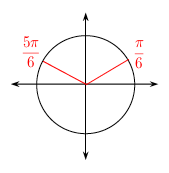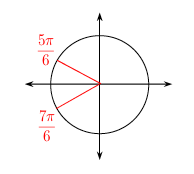### Home > INT3 > Chapter Ch12 > Lesson 12.1.2 > Problem12-25

12-25.

For each of the following equations, what are the solutions that lie in the domain $0\le x<2\pi$? Homework Help ✎

1. $2 \operatorname { sin } ( x ) - 1 = 0$

$\text{For the equation to be true, sin(x) must= }\frac{1}{2}.$

You can either draw a unit circle to find all possible solutions, or solve the equation graphically.1. $2 \operatorname { cos } ( x ) = - \sqrt { 3 }$

In what quadrants is cos(x) negative?1. $2 \operatorname { sin } ( x ) = \sqrt { 2 }$

$\text{When does sin(x)= }\frac{\sqrt{2}}{2}?$

1. $\operatorname { cos } ( x ) = 1$

Picture a unit circle. At what angle is the adjacent side the same length as the hypotenuse?